# Fraction calculator

The calculator performs basic and advanced operations with fractions, expressions with fractions combined with integers, decimals, and mixed numbers. It also shows detailed step-by-step information about the fraction calculation procedure. Solve problems with two, three, or more fractions and numbers in one expression.

## Result:

### 12/3 - 1/4 = 17/12 = 1 5/12 ≅ 1.4166667

Spelled result in words is seventeen twelfths (or one and five twelfths).

### How do you solve fractions step by step?

1. Conversion a mixed number 1 2/3 to a improper fraction: 1 2/3 = 1 2/3 = 1 · 3 + 2/3 = 3 + 2/3 = 5/3

To find a new numerator:
a) Multiply the whole number 1 by the denominator 3. Whole number 1 equally 1 * 3/3 = 3/3
b) Add the answer from previous step 3 to the numerator 2. New numerator is 3 + 2 = 5
c) Write a previous answer (new numerator 5) over the denominator 3.

One and two thirds is five thirds
2. Subtract: 5/3 - 1/4 = 5 · 4/3 · 4 - 1 · 3/4 · 3 = 20/12 - 3/12 = 20 - 3/12 = 17/12
For adding, subtracting, and comparing fractions, it is suitable to adjust both fractions to a common (equal, identical) denominator. The common denominator you can calculate as the least common multiple of both denominators - LCM(3, 4) = 12. In practice, it is enough to find the common denominator (not necessarily the lowest) by multiplying the denominators: 3 × 4 = 12. In the following intermediate step, it cannot further simplify the fraction result by canceling.
In other words - five thirds minus one quarter = seventeen twelfths.

#### Rules for expressions with fractions:

Fractions - simply use a forward slash between the numerator and denominator, i.e., for five-hundredths, enter 5/100. If you are using mixed numbers, be sure to leave a single space between the whole and fraction part.
The slash separates the numerator (number above a fraction line) and denominator (number below).

Mixed numerals (mixed fractions or mixed numbers) write as integer separated by one space and fraction i.e., 1 2/3 (having the same sign). An example of a negative mixed fraction: -5 1/2.
Because slash is both signs for fraction line and division, we recommended use colon (:) as the operator of division fractions i.e., 1/2 : 3.

Decimals (decimal numbers) enter with a decimal point . and they are automatically converted to fractions - i.e. 1.45.

The colon : and slash / is the symbol of division. Can be used to divide mixed numbers 1 2/3 : 4 3/8 or can be used for write complex fractions i.e. 1/2 : 1/3.
An asterisk * or × is the symbol for multiplication.
Plus + is addition, minus sign - is subtraction and ()[] is mathematical parentheses.
The exponentiation/power symbol is ^ - for example: (7/8-4/5)^2 = (7/8-4/5)2

#### Examples:

subtracting fractions: 2/3 - 1/2
multiplying fractions: 7/8 * 3/9
dividing Fractions: 1/2 : 3/4
exponentiation of fraction: 3/5^3
fractional exponents: 16 ^ 1/2
adding fractions and mixed numbers: 8/5 + 6 2/7
dividing integer and fraction: 5 ÷ 1/2
complex fractions: 5/8 : 2 2/3
decimal to fraction: 0.625
Fraction to Decimal: 1/4
Fraction to Percent: 1/8 %
comparing fractions: 1/4 2/3
multiplying a fraction by a whole number: 6 * 3/4
square root of a fraction: sqrt(1/16)
reducing or simplifying the fraction (simplification) - dividing the numerator and denominator of a fraction by the same non-zero number - equivalent fraction: 4/22
expression with brackets: 1/3 * (1/2 - 3 3/8)
compound fraction: 3/4 of 5/7
fractions multiple: 2/3 of 3/5
divide to find the quotient: 3/5 ÷ 2/3

The calculator follows well-known rules for order of operations. The most common mnemonics for remembering this order of operations are:
PEMDAS - Parentheses, Exponents, Multiplication, Division, Addition, Subtraction.
BEDMAS - Brackets, Exponents, Division, Multiplication, Addition, Subtraction
BODMAS - Brackets, Of or Order, Division, Multiplication, Addition, Subtraction.
GEMDAS - Grouping Symbols - brackets (){}, Exponents, Multiplication, Division, Addition, Subtraction.
Be careful, always do multiplication and division before addition and subtraction. Some operators (+ and -) and (* and /) has the same priority and then must evaluate from left to right.

## Fractions in word problems:

• Mother 7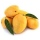Mother bought 18 fruits. 1/3 were pineapple and the rest  were mangoes . how many were mangoes
• Circular gardenAlice creates a circular vegetable garden. Tomatoes are planted in 1/3 of the circular garden, carrots are planted in 2/5 of the circular garden, and green peppers are planted in 1/10 of the circular garden. What fraction represents the remaining unplante
• Sum of 18Sum of two fractions is 4 3/7. If one of the fractions is 2 1/5 find the other one .
• Enrollees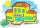The enrolment for this school year in Villahermosa integrated school is 276. If 1/3 are grade ten and are 1/4 grade seven, how many are grade eight and grade nine enrollees?
• Bucket 4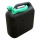The bucket has 5/8 gallons of water. The bucket tips over, and 7/12 gallon of water pours out. How much water is left in the bucket, in gallons, written as a fraction?
• PizzasBilly ate 1 1/4 pizzas and John ate 1 2/3 pizzas. How much more pizza did John eat than Billy?
• KevinKevin is baking brownies and needs peanuts.  The recipe calls for 1 and 2/3 cups of peanuts.  Kevin only has 5/6 of a cup of peanuts.  How many more cups of peanuts does Kevin need to have the correct amount?
• Two pizzasJacobs mom bought two whole pizzas. He ate 2/10 of the pizza and his dad ate 1 1/5. How much is left.
• RegroupingSubtract mixed number with regrouping: 11 17/20- 6 19/20
• Mrs. SusanMrs. Susan bought 1/8 m of curtain cloth. She used 3/5 m to make a curtain for the living room window. How many meters of cloth were not used?
• Players - baseball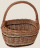There are 20 players on each of two baseball teams. If 2/5 of the players on team 1 miss practice and 1/4 of the players on team two miss practice, how many more players from team 1 missed practice then team 2?To three-eighths of one third, we add five quarters of one half and multiply the sum by four. How much will we get?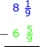Antonio is thinking of an improper fraction and a mixed fraction which have a sum of 5 5/12. The improper fraction is 8/3. What is the mixed number?